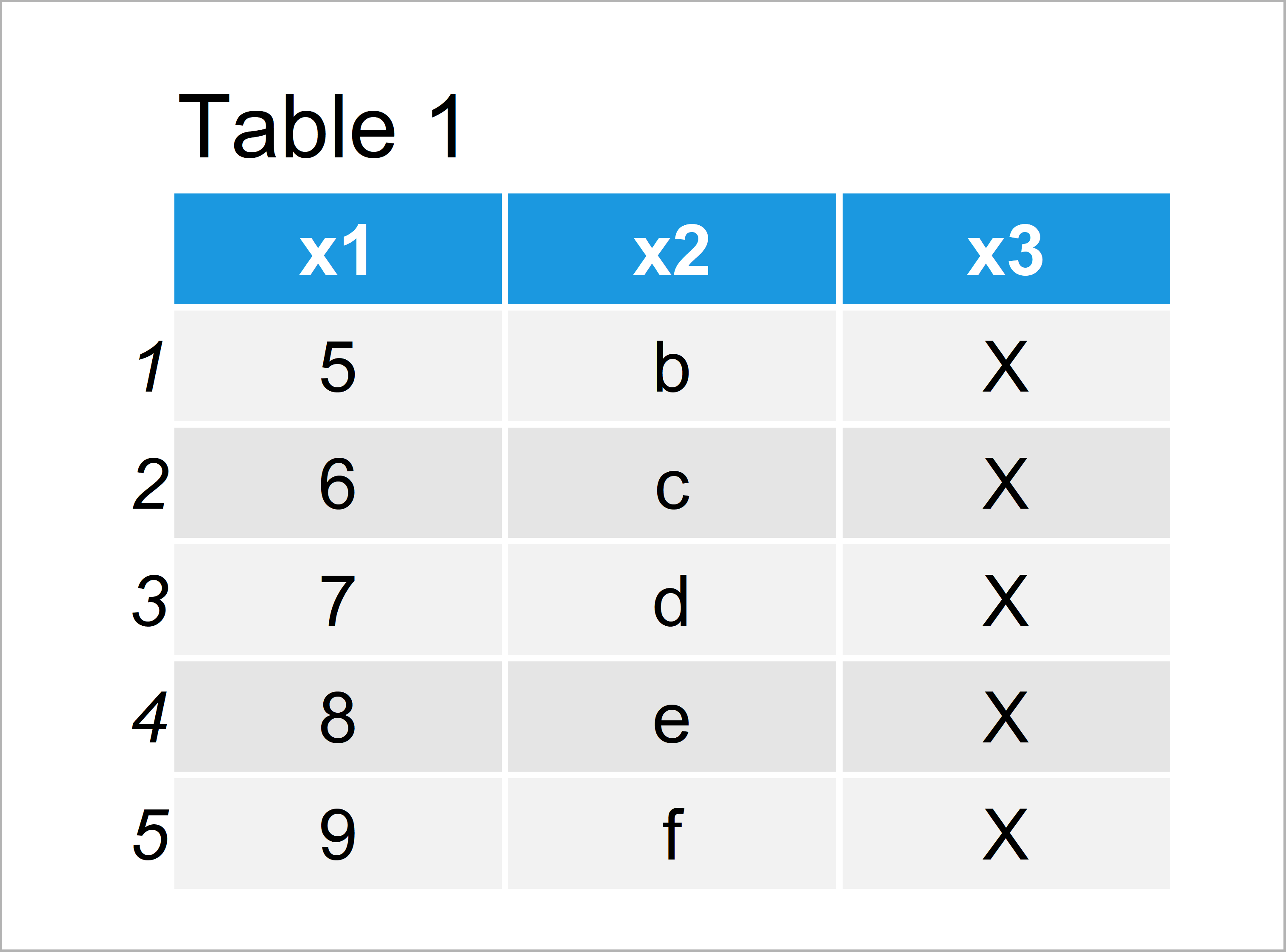# Convert Data Frame Columns to List Elements in R (2 Examples)

In this article you’ll learn how to convert data frame variables to list elements in the R programming language.

The tutorial consists of these contents:

Let’s do this.

## Creating Example Data

Consider the following example data:

```data <- data.frame(x1 = 5:9,         # Create example data
x2 = letters[2:6],
x3 = "X")
data                                 # Print example data```Table 1 shows the structure of our exemplifying data – It contains five rows and three columns.

## Example 1: Convert One Particular Variable to List Element

Example 1 explains how to set one specific variable of our data frame as list element of a new list in R.

For this, we can use the list function as shown below:

```my_list1 <- list(data\$x1)            # Convert one column to list element
my_list1                             # Print list
# []
#  5 6 7 8 9
#```

As you can see based on the previous output of the RStudio console, we have created a list with one list element. This list element consists of the values of the variable x1.

## Example 2: Convert Each Variable of Data Frame to List Element

In Example 2, I’ll explain how to set each column of a data frame as list element of a new list.

First, we have to create an empty list in R:

`my_list2 <- list()                   # Create empty list`

Next, we can use a for-loop to store each variable of our data frame as list element.

```for(i in 1:ncol(data)) {             # Using for-loop to add columns to list
my_list2[[i]] <- data[ , i]
}```

Next, we can modify the names of our list elements using the colnames function. In this example, we use the column names of our data frame as names of the list elements.

`names(my_list2) <- colnames(data)    # Rename list elements`

Finally, we can print our list to the RStudio console to see its structure:

```my_list2                             # Print list
# \$x1
#  5 6 7 8 9
#
# \$x2
#  "b" "c" "d" "e" "f"
#
# \$x3
#  "X" "X" "X" "X" "X"
#```

As you can see, each variable of our input data frame was converted to a new list element.

## Video & Further Resources

Do you need more information on the content of this article? Then you could watch the following video which I have published on my YouTube channel. I’m showing the content of this post in the video.

Please accept YouTube cookies to play this video. By accepting you will be accessing content from YouTube, a service provided by an external third party.If you accept this notice, your choice will be saved and the page will refresh.

Furthermore, you may want to read the other R posts on this website.

At this point you should have learned how to set a data frame column as a list element in R. If you have any additional questions or comments, let me know in the comments.

Subscribe to the Statistics Globe Newsletter